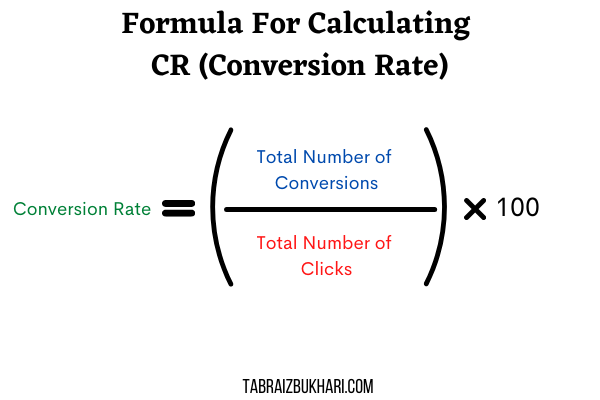Tabraiz Bukhari Conversion Rate Calculator

# Conversion Rate CalculatorYou can find a very easy-to-use conversion rate calculator below. You can find out the conversion rate of your ads campaigns by filling in the number of clicks and the number of conversions your ads have.

Conversion rate usually determines how well your ads are working. It tells you how many clicks on your ads or website are resulting in conversions. If your conversion rate is high, that means the audience is optimized. If the conversion rate is low then you need to change some metrics of your ads to get the right audience and better results.

## Conversion Rate Formula

To calculate conversion rate you need two metrics which are the total number of clicks and a total number of conversions. First, you divide the conversions by the number of clicks and then you multiply the outcome by 100. So basically the conversion rate is the percentage of people who are converting on your website.